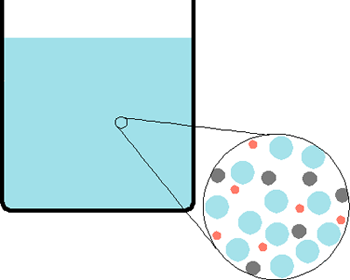# Reactions in Solution

A solution consists of two or more substances dissolved in a liquid form. Not to get confused with a mixture, which is heterogeneous--multiple substances exist in varying structures-- solutions are homogenous, which means that atoms of the solute are evenly dispersed throughout the solvent (ex. water, ethanol). Think of it as comparing a cup of (dissolved) sugar water and a cup of water with lego blocks in it. The solute is the substance dissolved in the solution, and the solvent is the substance doing the dissolving.

ex.

A solution of NaCl in water A mixture of lego blocks and waterNote: All solutions are mixtures but not all mixtures are solutions.

### Solvents

Water (H2O) is the most common solvent, used for dissolving many compounds or brewing coffee. Other common solvents include turpentine (a paint thinner), acetone (nail polish remover), and ethanol (used in some perfumes). Such solvents usually contain carbon and are called organic solvents. Solutions with water as the solvent are called aqueous solutions; they have special properties that are covered here.

### Solutes

Different chemical compounds dissolve in solutes in varying degrees. Some compounds, such as the strong acid hydrochloric acid (HCl), dissociate completely in solution into ions. Others, like the weak base ammonia (NH3), only partly dissociate. Yet other compounds like alcohol do not dissociate at all and remain compounds. Laboratory reactions often involve acids and bases, which are covered in more detail here.

Concentration

Concentration is the measure of the amount of solute in a certain amount of solvent. Knowing the concentration of a solution is important determining the strength of an acid or base (pH), among other things. When there is so much solute present in a concentration that it no longer dissolves, the solution is saturated.

Scientists often use molarity to measure concentration.

molarity = moles/Liter

Since reaction stoichiometry relies on molar ratios, molarity is the main measurement for concentration.

A less common unit for concentration is called molality.

Molality = moles/Kg of solvent

Scientists sometimes use molality to measure concentration because liquid volumes change slightly based on the temperature and pressure. Mass, however, stays the same and can be measured accurately using a balance. Commercial concentrated products are usually expressed in mass percent; such as commercial concentrated sulfuric acid, which is 93-98% H2SO4 by mass in water (Hill, Petrucci 116).

### Making a Solution

Solutions used in the laboratory are usually made from either solid solutes (often salts) or stock solutions.

To make a solution from solid solutes, first calculate how many moles of solute are in the desired solutions (using the molarity). Calculate the amount of solid you need in grams using the moles needed and the molar mass of the solute and weight out the needed amount. Transfer the solute to a container (preferably a volumetric flask, which most accurately measures volume of solution labeled on the flask) and add a small amount of solvent. Mix thoroughly to dissolve the solute. Once the solute has dissolved, add the remaining solvent to make the solution of the desired volume and mix thoroughly.

For example, to make 0.5 Liters of 0.5 molar NaCl:

1. Multiply the concentration (0.5 mols/Liters) by the volume of solution you want (0.5 Liters) to find the moles of NaCl you need.

0.5 moles/Liter * 0.5 Liters = 0.25 moles of NaCl

2. Multiply the moles of NaCl by its molar mass (58.44 g/mol) to find the grams of solute needed.

(0.25 moles NaCl)*(58.44 grams/mole) = 14.61 grams of NaCl

Making a solution of a certain concentration from a stock solution is called a dilution. When diluting a solution, keep in mind that adding a solvent to a solution changes the concentration of the solution, but not the amount of solute already present.

To dilute a solution with known concentration, first determine the number of moles of solute are in the solution by multiplying the molarity by the volume (in Liters). Then, divide by the desired molarity or volume to find the volume or concentration needed.

The equation to use is simply

M1V1 = M2V2

M1 and V1 are the concentration and volume of the original (stock) solution to dilute; M2 and V2 are the desired concentration and volume of the final solution.

## Solution Stoichiometry

For reactions that take place in solutions:

1. Calculate the moles of solute reacting by multiplying the concentration (molarity) by the volume of solution (Liters)
2. Determine the Limiting Reactant, if there is one